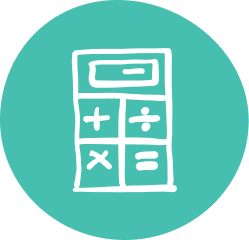### Quality Grade 12 Math Tutoring & Help

Oxford Learning®’s 12th Grade math tutoring program helps students extend their learning skills and mathematical comprehension.

Whether a student is aiming for university, college, or the workplace, Oxford Learning®’s Grade 12 math tutors will help students boost math skills and math grades. Students extend their math knowledge with Oxford Learning®’s Grade 12 math help program, helping them achieve better grades and develop skills for life. With our personalized approach to instruction, students focus on the areas that need the most attention, while developing learning skills they can rely on in post-secondary.

### Grade 12 Math Tutoring Subjects

##### Exponential & Logarithmic Functions
• Solve exponential and simple logarithmic equations arising from real-world problems
##### Trigonometric Functions
• Prove trigonometric identities
• Understand the meaning of radian measure
##### Polynomial & Rational Functions
• Solve polynomials
• Simple rational inequalities
##### Characteristics of Functions
• Model and reason with functions, including problems not solvable by standard algebraic techniques
##### Rate of Change
• Verify the connection between average rate of change over an interval and instantaneous rate of change at a point
##### Derivatives and Their Applications
• Make connections between the key features of a function and its first and second derivatives
##### Geometry & Algebra of Vectors
• Distinguish between a single linear equation and a system of two linear equations
##### Counting & Probability
• Combination
• Permutation
• Solve problems involving probability
##### Probability Distributions
• Determine standard deviations
• Understand continuous probability distributions
• Solve problems related to the real world
##### Organization of Data for Analysis
• Learn various sample techniques and data collection
##### Statistical Analysis
• Analyze, interpret, and draw conclusions from one-variable and two-variable data
• Understand how data is applied in the media and advertising industries
##### Culminating Data Management and Investigation
• Design and carry out a culminating investigation using the data management skills learned up to this point
##### Mathematical Models
• Evaluate powers of rational exponents
##### Personal Finance
• Annuities
• Mortgages
• Owning vs. renting
##### Geometry and Trigonometry
• Solve problems involving real-world geographical and trigonometric applications
##### Data Management
• Collect data
• Analyze data
• Summarize data